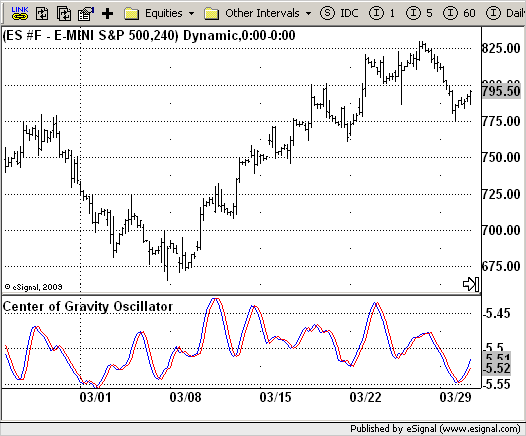# The Center Of Gravity

ICE Data Services -

Gravity.efs
EFSLibrary - Discussion Board

File Name: Gravity.efs

Description:
The Center Of Gravity

Formula Parameters:

• Length : 10

Notes:

This indicator identifies every major turning point without much lag.
This indicator is computed in a similiar way to the Ehlers filter.
The position of the balance point is the summation of the product of position within the observation window multiplied by the price at that position divided by the summation of prices across the window. The formula is:

`CG = SUM(x+1) * Price(i) / SUMPrice(i)`

In this formula "1" is added to the position count because it starts with the most recent price at zero, and multiplying the most recent price by that position count would remove it from the computation.

Gravity.efsEFS Code:

```/*********************************
Provided By:
eSignal (Copyright c eSignal), a division of Interactive Data
Formula Script (EFS) is for educational purposes only and may be
modified and saved under a new file name.  eSignal is not responsible
for the functionality once modified.  eSignal reserves the right
to modify and overwrite this EFS file with each new release.

Description:
The Center Of Gravity

Version:            1.0  05/05/2009

Formula Parameters:                     Default:
Length                              10

Notes:
This indicator identifies every major turning point without much lag.
This indicator is computed in a similiar way to the Ehlers filter.
The position of the balance point is the summation of the product of
position within the observation window multiplied by the price at that
position divided by the summation of prices across the window. The formula is:
CG = SUM(x+1) * Price(i) / SUMPrice(i)
In this formula "1" is added to the position count because it starts with
the most recent price at zero, and multiplying the most recent price by that
position count would remove it from the computation.
**********************************/
var fpArray = new Array();
var bInit = false;

function preMain() {
setStudyTitle("Center of Gravity Oscillator");
setCursorLabelName("Gravity", 0);
setCursorLabelName("Temp", 1);
setPriceStudy(false);
setDefaultBarFgColor(Color.red, 1);
setDefaultBarFgColor(Color.blue, 0);
var x = 0;
fpArray[x] = new FunctionParameter("Length", FunctionParameter.NUMBER);
with(fpArray[x++]) {
setLowerLimit(1);
setDefault(10);
}
}

var xGravity = null;

function main(Length) {
var nBarState = getBarState();
var nGravity = 0;
var nTemp = 0;
if (nBarState == BARSTATE_ALLBARS) {
if(Length == null) Length = 10;
}
if (bInit == false) {
xGravity = efsInternal("Calc_Gravity", Length);
bInit = true;
}
nGravity = xGravity.getValue(0);
nTemp =  xGravity.getValue(-1);
if (nTemp == null) return;
return new Array(nGravity, nTemp);
}

var bSecondInit = false;
var xHL2 = null;

function Calc_Gravity(Length) {
var nRes = 0;
var i = 0;
var nHL2 = 0;
var num = 0;
var denom = 0;
if (bSecondInit == false) {
xHL2 = hl2();
bSecondInit = true;
}
if (xHL2.getValue(-Length) == null) return;
for(i = 0; i < Length; i++) {
nHL2 = xHL2.getValue(-i);
num += (1 + i) * nHL2;
denom += nHL2;
}
if(denom != 0) 	nRes = - num / denom;
return nRes;
}```